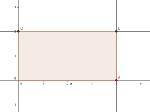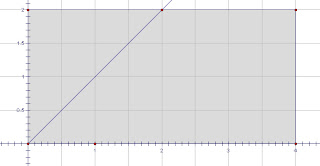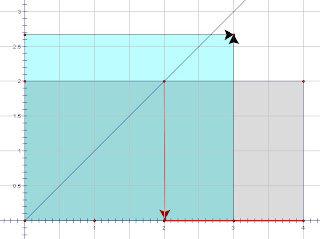## Wednesday 23 October 2013

### Some Geometry Notes on a Babylonian Square RootPhysical evidence exists that the Babylonians had a method of calculating the square root of some numbers as early as 2000 years before the birth of Christ. In the Yale collection there exist an artifact that shows the calculation of the square root of two to five decimal places accurately. One image of the item, called YBC7289, can be seen above. What may surprise many students is that the ancient Babylonian method seems to be the same as the method frequently taught in school text books. The method is frequently attributed to Heron (or Hero) because it appears in his Metrica, and is also called Newton’s method, but most students who know the method, know it as the "divide-and-average" method.

I was recently reminded of this while reading a nice post about it from Brent over at The Math Less Traveled. He did a nice job of showing why it worked...except.. It was all algebraic.. and I don't think the Babylonians had a very developed synthetic algebra at all. So I wondered how they might have convinced themselves of its authenticity by geometric methods.
This is a flight of whimsey, or maybe two, because I have no evidence they thought these ideas anymore than they thought algebraic ones, but it was a good exercise in geometry, kept me out of trouble for awhile, and that's not all bad....

So let's pretend our ancient Babylonian scholar is trying to reason out the square root of eight. He knows that means he is looking for the sides of a square with an area of eight. So.... he picks an unlikely starting point, and guesses four. Then he creates a rectangle with one side of four that has an area of eight.(I have kept these images small for space, but if you click on them, I think they can be expanded to accommodate somewhat better viewing)

Knowing full well that if he bisected the angle in a corner of a square, he would also bisect the opposite corner, he constructs the angle bisector (y=x in my image) and observes that it does NOT hit the opposite corner... a 2x4 rectangle is NOT a square..Now he drops a vertical line to the base of the rectangle (x-axis to we moderns) and can see the difference between his two rectangle sides... SO, let's just average the two sides and try again. (our Babylonian scholar would use his compass and bisect this segment)  We make our base equal to the average of 2 and 4 (I got 3) he sets his base equal to 3.Dividing eight by this new length, he creates another rectangle with an area of eight that has sides of 3 and 2 2/3, closer to equal than the last. Noticing that it is Still not a square, he constructs another base averaging 3 and 2 2/3 and repeats until the accuracy of the construction meets his needs.A second excursion of fantasy adopts a more modern idea that is too little known to students and teachers today. If you pick two points (a, a2) and (b, b2) on the curve y=x2, the line segment connecting them will cross the y-axis at the point (0, -ab)... This gives an easy way to find the square root of eight.  I constructed a point at (0,2) and used a horizontal line (easy on graph paper) to find the point (1.414,2), then the point (2,4) falls out on grid paper quite easily.  The line will now intersect the y-axis at the point x=0 and y= -sqrt(8). Of course a clever student might just draw a horizontal line at (0,8) and that would intersect at (sqrt(8), 8) also.

I wanted to use the property to illustrate what happens when we use the divide and average method. Working backwards, we can start with a point at (4, 16) and drew a line through (0,8) to the opposite side of the parabola it would intersect at (-2,4) [because -(-2)x4=8].

If our line through (0,8) was level, it would mean we had equal sides, and hence sides of a square of eight. when we average them (2 and 4 again) and plot a point on the new x value (x=3 in this case), we are at (3,9) (red)
Then another ray through (0,8) to the opposite side and we hit (8/3, 64/9)to give the other side of our second rectangle. Repeat as needed....
So two geometric approaches to the divide and average method of finding square roots. Hope they may be useful to someone.